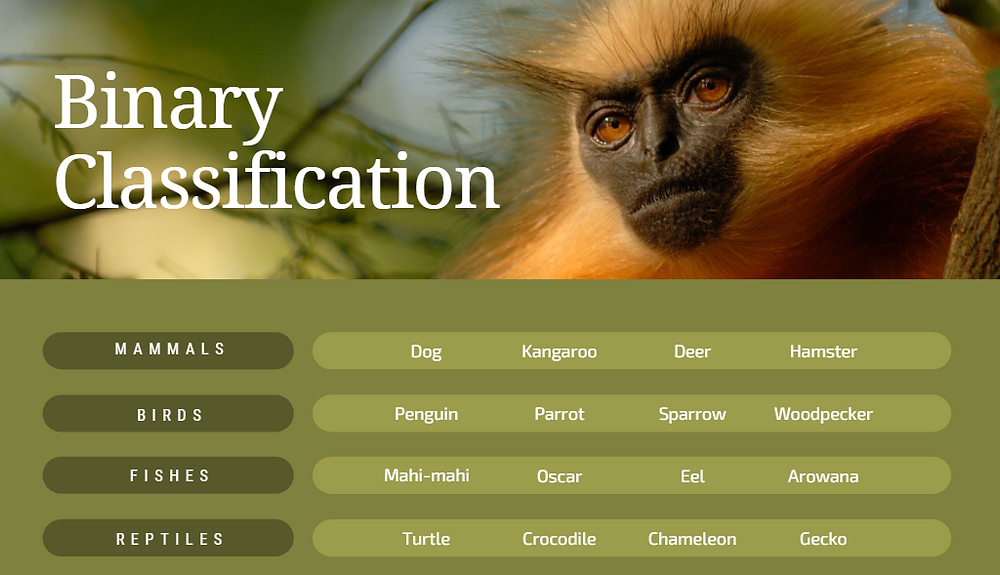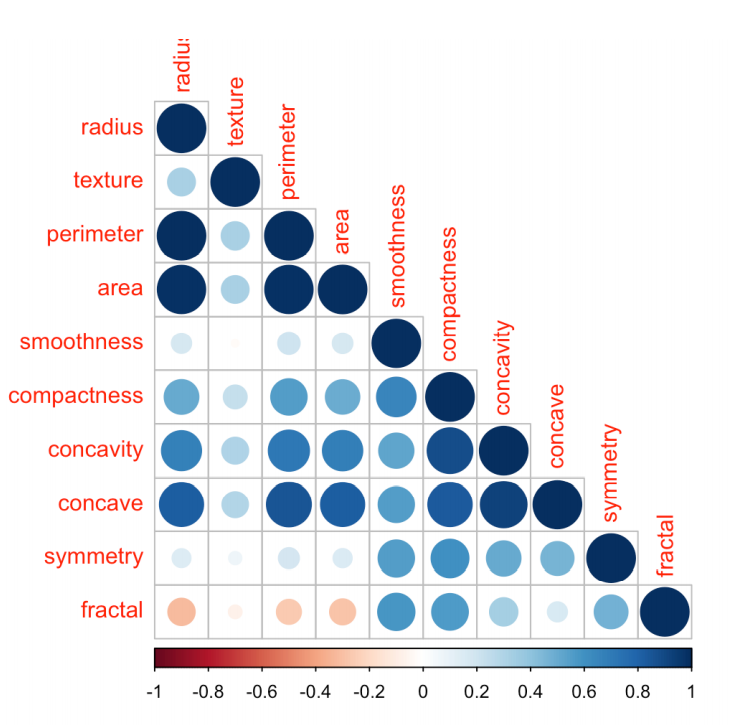Search

# Binary Classification Using R Programming | Machine Learning Homework HelpIn this project you will work through a binary classification problem using R. After completing this project, you will know:

1. How to work through a binary classification predictive modelling problem end-to-end.

2. How to use data transforms and model tuning to improve model accuracy.

3. How to identify when you have hit an accuracy ceiling and the point of diminishing returns on a project.

Problem Definition

For this project we are building a model that will predict whether a tumour is malignant (M) or benign (B), based on data from a study on breast cancer. The data set refers to 569 patients from a study on breast cancer, and it is publicly available at UCI (Machine Learning Repository):

https://archive.ics.uci.edu/ml/datasets/Breast+Cancer+Wisconsin+(Diagnostic). You can find the dataset in the Learning Material of this week on Blackboard.

We will use two distinct classification algorithms: logistic regression (typically used for binary classification), and Decision Trees, and compare their performance.

Data set

The data for this analysis refer to 569 patients from a study on breast cancer. The variables were computed from a digitized image of a breast mass and describe characteristics of the cell nucleus present in the image. Specifically, the variables are the following:

a. radius (mean of distances from centre to points on the perimeter)

b. texture (standard deviation of gray-scale values)

c. perimeter

d. area

e. smoothness (local variation in radius lengths)

f. compactness (perimeter^2 / area - 1.0)

g. concavity (severity of concave portions of the contour)

h. concave points (number of concave portions of the contour)

i. symmetry

j. fractal dimension (“coastline approximation” - 1)

k. type (tumor can be either malignant M or benign B)

```# Load Libraries
library(dplyr)
library(tidyr)
library(corrgram)
library(ggplot2)
library(ggthemes)
library(cluster)
library(caret)
library(ggplot2) ```

# Insert dataset into R

# Discard the id column as it will not be used in any of the analysis below med

<- med[, 2:12]

# change the name of the first column to diagnosis

colnames(med) <- "diagnosis"

Exploratory Data Analysis and Visualisations

Before using the machine learning algorithms for classification task, it is essential to have an overview of the dataset. Below there is a box-plot of each predictor against the target variable (tumour). The log value of the predictors used instead of the actual values, for a better view of the plot.

```# Create a long version of the dataset
med2 <- gather(med, "feature", "n", 2:11)
ggplot(med2)+  geom_boxplot(aes(diagnosis, log(n)))+
facet_wrap(~feature, scales = "free")+
labs(title = "Box-plot of all predictors(log scaled) per tumor type",
subtitle = "tumor can be either malignant -M- or benign -B-")+
theme_fivethirtyeight()+
theme(axis.title = element_text()) +
ylab("Predictor's log value") +
xlab('')```

Output:It seems that for most predictors the malignant level of tumour type has higher values than the benign level. Now let’s see if the predictors are correlated. Below there is a scatter-plot matrix of all predictors

```# Scatterplot matrix of all numeric variables
pairs(~., data = med[, sapply(med, is.numeric)], main = "Scatterplot Matrix of variables")```

Output:We can observe how there are some predictors that are strongly related, as expected, such as radius, perimeter, and area. A correlogram will serve us better and quantify all correlations.

```library(corrplot)
# Plot correlogram of numeric variables
corrplot(cor(med[,2:11]), type="lower", tl.srt = 90)```

Output:We can spot some less significant correlations, such as concave and concavity and compactness. Also concave against radius, perimeter, and area.

Making prediction using classification methods

In the first part of this analysis, the goal is to predict whether the tumour is malignant or benign based on the variables produced by the digitized image using classification methods. Classification tasks consists of identifying to which of a set of categories (sub-populations) a new observation belongs, on the basis of a training set of data containing observations (or instances) whose category membership is known. Thus, we need to develop a model that classifies (categorise) each tumour (case) to either malignant or benign. Classification will be performed with two different methods, Logistic Regression and Decision Trees.

Feature selection

It is important to use only significant predictors while building the prediction model. You do not need to use every feature at your disposal for creating an algorithm. You can assist the algorithm by feeding in only those features that are really important. Below there are some reasons for the feature selection:

• It enables the machine learning algorithm to train faster.

• It reduces the complexity of a model and makes it easier to interpret.

• It improves the accuracy of a model if the right subset is chosen.

• It reduces over-fitting.

In particular, I used the stepwise (forward & backward) logistic regression on the data, since the dataset is small. This method is computationally very expensive, so it is not recommended for very large datasets.

library(MASS)

# Create a logistic regression model

glm <- glm(diagnosis ~ ., family=binomial(link='logit'), data = med)

# Run the stepwise regression

both <- stepAIC(glm, direction = "both")

# This is the output in the R console

Start: AIC=168.13

diagnosis ~ radius_mean + texture_mean + perimeter_mean + area_mean + smoothness_mean + compactness_mean + concavity_mean + concave.points_mean + symmetry_mean + fractal_dimension_mean

Df Deviance AIC

- compactness_mean 1 146.14 166.14

- perimeter_mean 1 146.15 166.15

- fractal_dimension_mean 1 146.78 166.78

- concavity_mean 1 147.23 167.23

<none> 146.13 168.13

- symmetry_mean 1 148.44 168.44

- area_mean 1 151.63 171.63

- concave.points_mean 1 151.93 171.93

- smoothness_mean 1 152.42 172.42

- texture_mean 1 195.34 215.34

Step: AIC=166.14 diagnosis ~ radius_mean + texture_mean + perimeter_mean + area_mean + smoothness_mean + concavity_mean + concave.points_mean + symmetry_mean + fractal_dimension_mean

----

----

Logistic Regression

Logistic regression is a parametric statistical learning method, used for classification especially when the outcome is binary. Logistic regression models the probability that a new observation belongs to a particular category (or class). To fit the model, a method called maximum likelihood is used. Below there is an implementation of logistic regression.

# Create a vector with the 70% of the dataset with respect to diagnosis variable

set.seed(1)

inTrain = createDataPartition(med\$diagnosis, p = .7)[]

# Assign the 70% of observations to training data

training <- med[inTrain,]

# Assign the remaining 30 % of observations to testing data

testing <- med[-inTrain,]

# Build the model

glm_model <- glm(diagnosis~., data = training, family = binomial)

## Warning: glm.fit: fitted probabilities numerically 0 or 1 occurred

summary (glm_model)

# This is the output in the R console

Call:

glm(formula = diagnosis ~ ., family = binomial, data = training)

Deviance Residuals:

Min 1Q Median 3Q Max

-1.96003 -0.11469 -0.01671 0.00085 2.77666

Coefficients:

Estimate Std. Error z value Pr(>|z|)

(Intercept) -24.77270 11.81054 -2.098 0.03595 *

texture_mean 0.47942 0.09617 4.985 6.19e-07 ***

area_mean 0.05177 0.02100 2.465 0.01369 *

smoothness_mean 137.67123 32.23104 4.271 1.94e-05 ***

concavity_mean 14.51851 4.96897 2.922 0.00348 **

symmetry_mean 35.44872 14.61003 2.426 0.01525 *

---

Signif. codes: 0 ‘***’ 0.001 ‘**’ 0.01 ‘*’ 0.05 ‘.’ 0.1 ‘ ’ 1

(Dispersion parameter for binomial family taken to be 1)

Null deviance: 527.28 on 398 degrees of freedom Residual deviance: 93.25 on 392 degrees of freedom

AIC: 107.25

Number of Fisher Scoring iterations: 9

By looking at the summary output of the logistic regression model we can see that almost all coefficients are positive, indicating that higher measures mean higher probability of a malignant tumour.

An important step here is to evaluate the predicting ability of the model. Because the model’s predictions are probabilities, we must decide the threshold that will split the two possible outcomes. At first, I’ll try the default threshold of 0.5. Below there is a confusion matrix of with predictions using this threshold.

options(scipen=999)

# Apply the prediction

prediction <- predict(glm_model, newdata= testing, type = "response") prediction <- ifelse(prediction > 0.5, "M", "B")

# Check the accuracy of the prediction model by printing the confusion matrix print(confusionMatrix(as.factor(prediction), testing\$diagnosis), digits=4)

# This is the output in the R console

Confusion Matrix and Statistics

Reference

Prediction B M

B 102 6

M 5 57

Accuracy : 0.9353

95% CI : (0.8872, 0.9673)

No Information Rate : 0.6294

P-Value [Acc > NIR] : <0.0000000000000002

Kappa : 0.8608

Mcnemar's Test P-Value : 1

Sensitivity : 0.9533

Specificity : 0.9048

Pos Pred Value : 0.9444

Neg Pred Value : 0.9194

Prevalence : 0.6294

Detection Rate : 0.6000

Detection Prevalence : 0.6353

Balanced Accuracy : 0.9290

'Positive' Class : B

The overall accuracy of the model is 93.53 % (7.47 % error rate). But in this specific case we must distinguish the different types of error. In other words, there are two types of error rate, type I and type II errors. In our case these are: type II error = 4.67% (sensitivity) and type I error = 9.52% (specificity). Type I error means that a benign tumour is predicted to be malignant and type II error when a malignant tumour is predicted to be benign. Type II error is more expensive, and we must find ways to eliminate it (even if it increases type I error). Below I increased the threshold to 0.8, which changed the prediction model.